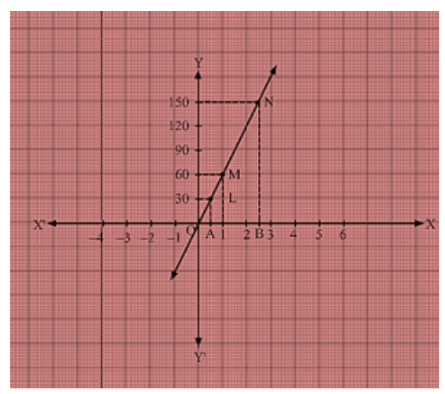# Aarushi was driving a car with uniform speed of 60 km/h.

Question:

Aarushi was driving a car with uniform speed of 60 km/h. Draw distance-time graph From the graph, find the distance travelled by Aarushi in

(i) 5/2 Hours

(ii) 1/2 Hour

Solution:

Aarushi is driving the car with the uniform speed of 60 km/h. We represent time on X-axis and distance on Y-axis Now, graphicallyWe are given that the car is travelling with a uniform speed 60 km/hr.

This means car travels 60 km distance each hour.

Thus the graph we get is of a straight line.

Also, we know when the car is at rest, the distance travelled is 0 km, speed is 0 km/hr and the time is also 0 hr.

Thus, the given straight line will pass through O(0, 0) and M(1, 60).

Join the points 0 and M and extend the line in both directions.

Now, we draw a dotted line parallel to y-axis from x = 12 that meets the straight line graph at L from which we draw a line parallel to x-axis that crosses y-axis at 30.

Thus, in 12 hr, distance travelled by the car is 30 km.

Now, we draw a dotted line parallel to y-axis from x = 5/2  that meets the straight line graph at N from which we draw a line parallel to x-axis that crosses y-axis at 150.

Thus, in 5/2 hr, distance travelled by the car is 150 km.

(i) Distance = Speed × Time Distance travelled in 5/2 hours is given by

Distance = 60 × 5/2, Distance = 150 Km

(ii) Distance = Speed × Time Distance travelled in 12 hour is given by Distance = 60 × 5/2

Distance = 30 km